Directional region control of the thermal fractal diffusion of a space body*
School of Control Science and Engineering, Shandong University, Jinan 250061, China
School of Mechanical and Electrical Engineering, Shandong University at Weihai, Weihai 264209, China

Corresponding author. E-mail: sunj@sdu.edu.cn

*Project supported by the National Natural Science Foundation of China (Grant Nos. 61273088, 61473173, and 61473174).

Abstract

We present a directional region control (DRC) model of thermal diffusion fractal growth with active heat diffusion in three-dimensional space. This model can be applied to predict the space body heat fractal growth and study its directional region control. When the nonlinear interference term and the inner heat source term are generalized functions, the relationship between the particle aggregation probability and the interference terms can be obtained using the norm theory. We can then predict the aggregation form of particles in different regions. When the nonlinear interference terms in the model are expressed as a trigonometric function and its composite function, our simulations show that the DRC method of thermal fractal diffusion is effective and has reference value for the directional control of actual fractal growth systems.

Keyword: 05.45.Df; 61.43.Hv; thermal diffusion; fractal growth; directional region control
1. Introduction

Fractal geometry theory plays an important role in the nonlinear field. The diffusion limited aggregation (DLA) model proposed by Witten and Sander, which explains the far-from equilibrium natural phenomenon, is an especially hot research topic. Various improved DLA models have been proposed to better explain specific growth processes such as dendritic growth,  electro-deposition,  and fluid displacement. The DLA model allows researchers to obtain the features of fractal growth and derive the morphology control theory under some two-dimensional growth conditions. However, natural fractal growth is conducted in the three-dimensional space and the actual system is usually expected to control the fractal growth form in a fixed region, such as crystal growth occurring along specified axes, non-uniform bacterial colony growth, and particles aggregating along a certain orientation. At present, the orientation restriction issue for fractal growth is usually addressed only in experimental research,  and it is seldom tackled theoretically. Meanwhile, many external environmental factors may interfere with the process of fractal growth, which makes it complicated and difficult to predict the morphology of fractal growth. Therefore, the research on the directed prediction and control of three-dimensional fractal growth is of great significance.

We consider a space body in a real environment and provide a directional region control model for the thermal fractal diffusion system. The quantitative relationship between the particle aggregation probability and the interference term is obtained using the norm theory, in which the nonlinear interference term and the internal heat source term are generalized functions. Under certain conditions, the source term can suppress the nonlinear interference term, meaning that the source term controls the growth particles to aggregate in a different direction or region. This situation is called directional region control (DRC) in this paper. We predict the aggregation morphology of particles in differently directed regions, and achieve the DRC of space body thermal diffusion growth.

2. Space body thermal diffusion system

Considering the thermal diffusion of a space body W (as shown in Fig.  1) in an actual complex environment, we take the thermal diffusion process as a distributed parameter system meeting the boundary conditions,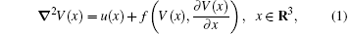where V(x) refers to the space body W ’ s temperature (the probability of thermal diffusion) at certain point x, and u(x) refers to the density of the inner heat resource. The f is a nonlinear function, referred to as the forced term in physics; u(x) is a quantitative initial condition, referred to as the source term. By assuming G = u(x) + f (V(x), V(x)/x), both the nonlinear interference term f (V(x), V(x)/x) and the source term u(x) can be regarded as a part of the environmental interference term G, which is assumed to be an arbitrary function. Figure  2 shows the thermal fractal diffusion aggregation cluster without environmental interference.

 Figure Option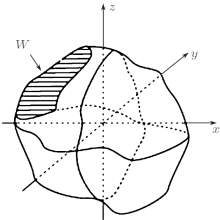Fig.  1. A space body W.

 Figure Option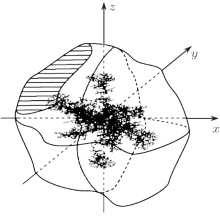Fig.  2. The thermal diffusion of the space body W (f = 0, u = 0).

Generalized functions can be regarded as continuous linear functionals of function space and are applied extensively in mathematics, physics, mechanics, and engineering. Let us assume that both f (V(x), V(x)/x) and u(x) are generalized functions, and that these generalized functions are all elements in a basic function space K. In general, it is very difficult to find an analytical solution to the nonlinear partial differential equation (1). By studying the weak solution to system (1), we discuss the quantitative relationship between the particle aggregation probability and the environmental interference term G.

3. Directional region control of a space body thermal fractal diffusion system

Definition 1 (L2 space) Assume that Ω is a bounded domain of R3. We use L2(Ω ) to express the function class consisting of all the measurable functions V(x) which are defined in Ω and meet Ω | V(x)| 2 dx < ∞ . The L2(Ω ) is a vector space with norms defined as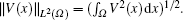Definition 2 (Sobolev space Hk) Assume that Ω is a bounded domain of R3. We use Hk(Ω ) to express the function class consisting of all the measurable functions which are defined in Ω and meet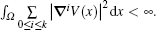The Hk(Ω ) is a vector space with norms defined as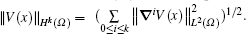Definition 3 Assume that a(V, v) is a bilinear functional in Hilbert space H, i.e., V and v are both linear.

(i) a(V, v) is bounded, if there is a positive constant M ≥ 0, such that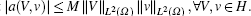(ii)| a(V, v)| is compulsory, if there is a positive constant δ > 0, such that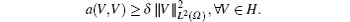Now we can consider the existence and uniqueness of the weak solution of the following system: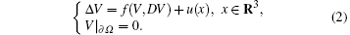Theorem 1 Assume that u(x) ∈ L2(Ω ), f (V, DV) ∈ L2(Ω ). There exists a unique weak solution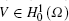to system (2), and the following estimation exists: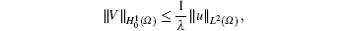where λ is a positive constant.

Proof Denote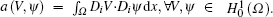Obviously, a(V, ψ ) is bilinear. First, we prove the boundedness of a(V, ψ ),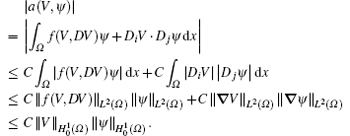Next, due to the ellipticity condition, we have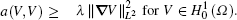So a(V, ψ ) is compulsory. Let us prove that F(ψ ) = Ω uψ dx is a bounded functional in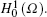. The F(ψ ) is linear. According to the Hö lder inequality, we haveTherefore, according to the Lax– Milgram Theorem,  there exists a uniquesuch that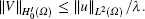. Thus,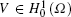is the only weak solution to system  (2).

To improve the regularity of the weak solution for system  (2), we introduce the difference operator. If V(x) is a function defined on R3, then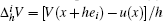where ei is the unit vector in the direction of xi and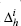is the difference operator along the direction of xi . Next, we introduce some of the basic properties of the difference operator.

Proposition 1 We have the following basic properties of the difference operator.

(i) The adjoint operator of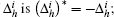for any integral functions, f (x), g(x) ∈ L2(Ω ), there is an integral equation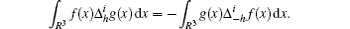(ii) The differential operator and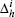are exchangeable; for any weak differentiable function ϕ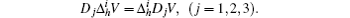(iii) The difference of products can be expressed as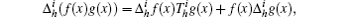where Ti is the shift operator in the direction of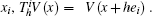Proposition 2 Assume that Ω R3 is a region.

(i) Assume that VH1(Ω ), Ω 1 ⊂ ⊂ Ω . For| h| > 0, when sufficiently small,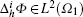, giving the following estimation formula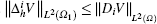(ii) Assume that V ⊂ L2(Ω ) and there exists a constant K such that Ω 1 ⊂ ⊂ Ω . When| h| > 0 is small enough,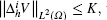where K is unrelated to h. Then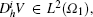giving the following estimation formula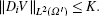We can obtain Theorem 2 using the basic properties of the difference operator.

Theorem 2 If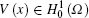) is the weak solution of system (2) and Ω 1 ⊂ ⊂ Ω , then VH2 (Ω 1) and we have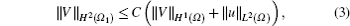where C is a positive constant that only depends on the distance dist {Ω 1, Ω } from Ω 1 to Ω .

Proof Supposing Ω 1Ω , we note that d = dist {Ω 1, Ω }/4. The cut-off function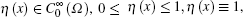dist{suppη , Ω } ≥ 2d.

Based on the definition of the weak solution and the density of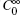in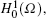we conclude that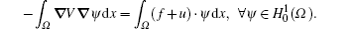If h meets the condition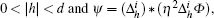then dist{suppψ , Ω } ≥ d, and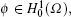therefore,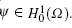Substituting ψ into the above integral equation, we can obtain the following formula: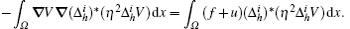By applying the exchangeability of the difference operator and the differential operator, and the relationship between the difference operator and its adjoint operator, the above integral relationship can be rewritten as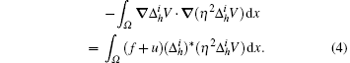The following formula is obtained from formula (4) by calculation: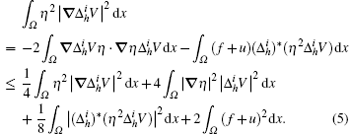For further calculations, we need to estimate the third term on the right side of formula  (5). From Proposition 1, we conclude that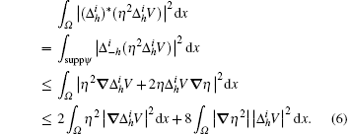Substituting formula (6) into formula (5), we obtain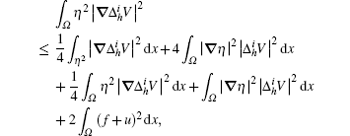and then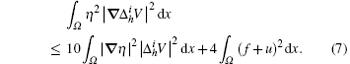Next, if we estimate the first term in formula (7) by using the cut-off function and Proposition 1, then we have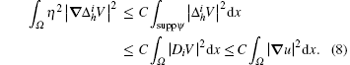Substituting formula (8) into formula (7), we conclude that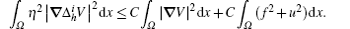Based on the above formula and using the cut-off function, we have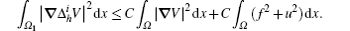Based on Proposition 2, we can conclude that formula (3) is correct. The proof is completed.

4. Simulation of the thermal fractal diffusion in directional regions

Based on Theorem 1, we can conclude that when f (V(x, y, z), DV(x, y, z) ∈ L2(Ω ), u(x, y, z) ∈ L2(Ω ), and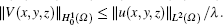The source term u(x) can restrain the nonlinear influence of the generalized function term f (V(x), V(x)/x) on the thermal fractal diffusion. This allows the thermal particle to be aggregated in different directions or regions, and achieves directed control.

By assuming that u(x) is a constant k, f(V(x), V(x)/x) = a sin2(V(x)), and f(V(x), V(x)/x) = bV(x)2 + c sin(V(x)), the control region H of the source item u(x) can be taken as a one-eighth space, one half space, cuboid region, spherical domain, or sectional region.

The thermal diffusion of a space body is a random process induced by a temperature gradient. In our recent work,  the thermal diffusion fractal growth was simulated. The growth-probability function of every possible aggregation point has a nonlinear relationship with the temperature gradient. With the environmental disturbance, the boundary condition V(x) is set to be zero at any point on the aggregation surface, and for the boundary at infinity, V(x) is set to be one, which provides a stable particle flow. By modifying the movement-boundary conditions of the mathematical model, the temperature gradient can be calculated from the Poisson equation. Furthermore, by using the roulette wheel selection algorithm, the particle aggregation location can be determined.

With the environmental interference considered, the thermal diffusions in different regions are simulated by using the simulation method in the literature. As shown in Figs.  2– 5, the interference source item controls the aggregation of the thermal particles in different directions. This result shows that our control method is valid.

A fractal dimension is an index for characterizing fractal patterns or sets by quantifying their complexity as a ratio of the change in detail to the change in scale. It has also been considered as a measure of the space-filling capacity of a pattern, which tells how a fractal scales differently from the space it is embedded in. As the thermal diffusion system of a space point source has an obvious central point, the Sandbox method is used to calculate the fractal dimension (Df) of the thermal aggregation cluster with different interference actions. Figure 6 shows the comparison between the thermal diffusion fractal dimensions of different sectional control regions. Figure  5 shows the results predicted by our DRC model for spherical domain, where the spherical interference control region is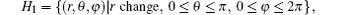and the sectional control region is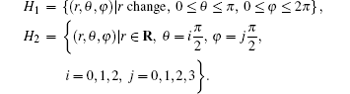According to Fig.  6, the aggregations of thermal particles based on the spherical control region are more complex than that controlled in different directions in addition to the spherical domain. Although they share the same control spherical domain, the directed region control beyond spherical domain determines the growth direction and also reduces the growth complexity. Figure  6 also shows that the fractal dimensions of clusters aggregated in the multidirectional region are larger than those of clusters aggregated in the unidirectional region for the same spherical domain. This indicates that the complexity of thermal aggregation clusters increases along with the increase in multidirectional control.

 Figure Option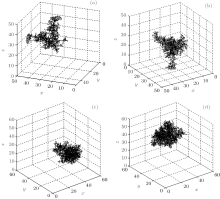Fig.  3. Thermal fractal diffusion in different interference space regions: (a) 0 ≤ θ ≤ π /2, 0 ≤ φ ≤ π /2, (b) π /2 ≤ θ ≤ π , π ≤ φ ≤ 3π /2, (c) π /2 ≤ θ ≤ π , 3π /2 ≤ φ ≤ 2π , (d) 0 ≤ θ ≤ π /2, 0 ≤ φ ≤ π .

 Figure Option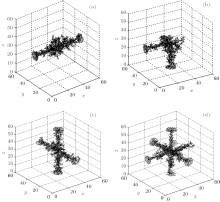Fig.  4. Thermal fractal diffusion under control conditions in differently directed regions: (a) θ = π /2, φ = jπ /2, j = 0, 2, (b) θ = π ; θ = π /2, φ = π , (c) θ = iπ /2, i = 0, 2; θ = π /2, φ = jπ /2, j= 1, 3, (d) θ = iπ /2, i = 0, 2; θ = π /2, φ = jπ /2, j = 0, 1, 2, 3.

 Figure Option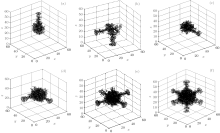Fig.  5. Thermal fractal diffusion with variation of the spherical domain and sectional region: (a) r ≤ 8; θ = 0, (b) r ≤ 8; θ = π ; θ = π /2, φ = jπ /2, j = 2, 3, (c) r ≤ 9; θ = π /2, φ = 3π /2, (d) r ≤ 10; θ = π /2, φ = jπ /2, j = 2, 3, (e) r ≤ 11; θ = π /2, φ = jπ /2, j = 0, 1, 2, 3, (f) r ≤ 14; θ = iπ /2, i = 0, 2; θ = π /2, φ = jπ /2, j = 0, 1, 2, 3.

 Figure Option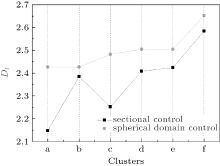Fig.  6. The fractal dimensions for the thermal diffusion clusters in Fig.  5 under variant spherical domain controls, and those under variant sectional controls.

5. Conclusion

We have presented a DRC model of thermal fractal diffusion with actual environmental interference. Based on the norm theory, the control analysis of this model is given. When the nonlinear interference terms and the inner heat source terms are generalized functions, the quantitative relationship between the particle aggregation probability and the disturbance term can be obtained using the norm theory. Our simulations show that this DRC model for the thermal diffusion fractal growth of a space body is valid. This paper addresses only the generalized form of the disturbance term in L2 space. If the generalized function belongs to the space Lp(p ≥ 1), then the DRC of space fractal growth will be of universal significance. The more complex theoretical structure will be subject to greater change and we will address the topic in a separate paper.

Reference
 1 Wang L and Zheng D Z 2000 Control Theory and Applications 17 139(in Chinese) [Cited within:1] [CJCR: 0.555] 2 Sun J, Liu S T and Qiao W 2011 Acta Phys. Sin. 60 70510(in Chinese) [Cited within:1] [JCR: 1.016] [CJCR: 1.691] 3 Wang X Y and Jin T 2013 Nonlinear Dynamics 73 843 DOI:10.1007/s11071-013-0836-5 [Cited within:1] [JCR: 3.009] 4 Wang X Y and Yu X J 2007 Applied Mathematics and Computation 189 1186 DOI:10.1016/j.amc.2006.12.002 [Cited within:1] [JCR: 1.349] 5 Wang X Y, Jia R H and Zhang Z F 2009 Applied Mathematics and Computation 210 107 DOI:10.1016/j.amc.2008.12.017 [Cited within:1] [JCR: 1.349] 6 Wang X Y and Wang Y X 2011 Chin. Phys. B 20 104202 DOI:10.1088/1674-1056/20/10/104202 [Cited within:1] [JCR: 1.148] [CJCR: 1.2429] 7 Yu S M and Chen G 2012 Communications in Nonlinear Science and Numerical Simulation 17 2617 DOI:10.1016/j.cnsns.2011.10.001 [Cited within:1] [JCR: 2.773] 8 Yu S M and Yu Z D 2008 Acta Phys. Sin. 57 6859(in Chinese) [Cited within:1] [JCR: 1.016] [CJCR: 1.691] 9 Yu S M, Lin Q H and Qiu S S 2004 Acta Phys. Sin. 53 2084(in Chinese) [Cited within:1] [JCR: 1.016] [CJCR: 1.691] 10 Witten T A and Sand er L M 1981 Phys. Rev. Lett. 47 1400 DOI:10.1103/PhysRevLett.47.1400 [Cited within:1] [JCR: 7.943] 11 Witten T A and Sand er L M 1983 Phys. Rev. B 27 5686 DOI:10.1103/PhysRevB.27.5686 [Cited within:1] 12 Suemi R R and Antonio S H 2013 Physica A: Statistical Mechanics and its Applications 392 3316 DOI:10.1016/j.physa.2013.03.044 [Cited within:1] [JCR: 1.676] 13 Suemi R R and Roberto M 2014 Chaos Soliton. Fract. 66 31 DOI:10.1016/j.chaos.2014.05.006 [Cited within:1] 14 Kolesnikov N N and Borisenko E B 2008 Journal of Crystal Growth 310 3287 DOI:10.1016/j.jcrysgro.2008.04.018 [Cited within:1] [JCR: 1.552] 15 Argoul F and Arneodo A 1988 Phys. Rev. Lett. 61 2558 DOI:10.1103/PhysRevLett.61.2558 [Cited within:1] [JCR: 7.943] 16 Shiny Kamz S H 2010 Computer Graphics Forum 29 675 DOI:10.1111/j.1467-8659.2009.01637.x [Cited within:1] [JCR: 1.638] 17 Shen S L 2008 Analysis and Control of Diffusion Limited Aggregation Growth Ji Nan Shand ong University(in Chinese) [Cited within:1] 18 Zhang L 2011 Control and Application of Fractal Growth Ji Nan Shand ong University(in Chinese) [Cited within:1] 19 Katyal N, Banerjee V and Puri S 2014 Journal of Quantitative Spectroscopy and Radiative Transfer 146 290 DOI:10.1016/j.jqsrt.2014.01.017 [Cited within:1] [JCR: 2.38] 20 He Q J and Huang Z L 2007 Journal of Crystal Growth 300 460 DOI:10.1016/j.jcrysgro.2006.11.226(in Chinese) [Cited within:1] [JCR: 1.552] 21 Dias E O 2013 Phys. Rev. E 88 063007 DOI:10.1103/PhysRevE.88.063007 [Cited within:1] [JCR: 2.313] 22 Evans P, Pemble M E and Sheel D W 2006 Chemistry of Materials 18 5750 DOI:10.1021/cm060816k [Cited within:1] [JCR: 8.238] 23 Qiao W, Liu S T and Sun J 2014 Control Theory & Applications 31 361(in Chinese) [Cited within:3] 24 Qi M Y and Wu F T 1999 Generalized Functions and Equations of Mathematical Physics Beijing Higher Education Press(in Chinese) [Cited within:1] 25 Lv H X and Wang T M 2011Applied Functional Analysis (Da Lian: Dalian University of Technology Press) (in Chinese) [Cited within:1] 26 Falconer K 2003 Fractal Geometry New York Wiley [Cited within:1] [JCR: 0.36] 27 Qiao W, Liu S T and Sun J 2014 Mathematical Problems in Engineering 2014 672547 DOI:10.1155/2014/672547 [Cited within:1] [JCR: 1.383]